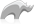##### [ bytes ]

Type

The bytes type represents a sequence of 8-bit elements. Bits can be imported from integers in various sized increments. For example, 8, 16, 32 or 64 bits can be imported at a time. A bytes object also permits its bits to be exported. Bytes objects maintain an internal position indicating where in the series of bits the next export will occur.

Bytes objects also permit string objects to be imported or exported using any valid character encoding. In this form, the [Bytes] type requires a string object to import as the first parameter, and an optional second parameter is a string that represents the encoding into which the string should be converted. If the second parameter is omitted, then the default encoding used is UTF-8.

• Syntax
• Methods
• Traits
``````local(Local Name) = bytes(Integer)
local(Local Name) = bytes(String Object)
local(Local Name) = bytes(String Object, Encoding String Value)``````
###### Has methods:
• `bytes->oncompare(p0::bytes)`
• `bytes->oncompare(s::string)`
• `bytes->setsize(p0::integer)`
• `bytes->append(p0::bytes)`
• `bytes->append(rhs::string)`
• `bytes->private_find(p0::bytes, p1::integer, p2::integer, p3::integer, p4::integer)`
• `bytes->reserve(p0::integer)`
• `bytes->getrange(p0::integer, p1::integer)`
• `bytes->sub(p0::integer, p1::integer)`
• `bytes->sub(pos::integer)`
• `bytes->private_setrange(p0::bytes, p1::integer, p2::integer, p3::integer)`
• `bytes->importas(p0::string, p1::string)`
• `bytes->import8bits(p0::integer)`
• `bytes->import32bits(p0::integer)`
• `bytes->import64bits(p0::integer)`
• `bytes->import16bits(p0::integer)`
• `bytes->importbytes(p0::bytes)`
• `bytes->importpointer(p0::integer, p1::integer)`
• `bytes->export8bits()`
• `bytes->export16bits()`
• `bytes->export32bits()`
• `bytes->export64bits()`
• `bytes->exportbytes(p0::integer)`
• `bytes->exportsigned8bits()`
• `bytes->exportsigned16bits()`
• `bytes->exportsigned32bits()`
• `bytes->exportsigned64bits()`
• `bytes->trim()`
• `bytes->marker()`
• `bytes->marker=(p0::integer)`
• `bytes->remove(p0::integer, p1::integer)`
• `bytes->remove()`
• `bytes->swapbytes()`
• `bytes->size()`
• `bytes->length()`
• `bytes->encodeurl(p0::boolean)`
• `bytes->encodeurl()`
• `bytes->decodeurl()`
• `bytes->encodebase64()`
• `bytes->decodebase64()`
• `bytes->encodeqp()`
• `bytes->decodeqp()`
• `bytes->encodemd5()`
• `bytes->encodehex()`
• `bytes->decodehex()`
• `bytes->uncompress()`
• `bytes->compress()`
• `bytes->detectcharset()`
• `bytes->bestcharset(p0::string)`
• `bytes->crc()`
• `bytes->asString()`
• `bytes->oncreate(initial::integer)`
• `bytes->oncreate(copy::bytes)`
• `bytes->oncreate(import::string)`
• `bytes->oncreate(import::string, ENCODING::string)`
• `bytes->oncreate(import)`
• `bytes->importString(s::string, enc::string = ?)`
• `bytes->+(rhs::bytes)`
• `bytes->+(rhs::string)`
• `bytes->find(find::bytes, position::integer = ?, length::integer = ?, patPosition::integer = ?, patLength::integer = ?)`
• `bytes->find(find::string, position::integer = ?, length::integer = ?, patPosition::integer = ?, patLength::integer = ?)`
• `bytes->contains(find)`
• `bytes->get(position::integer)`
• `bytes->beginsWith(find::string)`
• `bytes->beginsWith(find::bytes)`
• `bytes->endsWith(find::string)`
• `bytes->endsWith(find::bytes)`
• `bytes->padLeading(tosize::integer, with::bytes)`
• `bytes->padLeading(tosize::integer)`
• `bytes->padLeading(tosize::integer, s::string)`
• `bytes->padTrailing(tosize::integer, with::bytes)`
• `bytes->padTrailing(tosize::integer)`
• `bytes->padTrailing(tosize::integer, s::string)`
• `bytes->setrange(what::bytes, where::integer = ?, whatStart::integer = ?, whatLen::integer = ?)`
• `bytes->split(find::string)`
• `bytes->split(find::bytes)`
• `bytes->removeleading(find::bytes)`
• `bytes->removetrailing(find::bytes)`
• `bytes->replace(find::bytes, replace::bytes)`
• `bytes->replace(find::string, replace::string)`
• `bytes->exportAs(ENCODING::string = ?)`
• `bytes->exportString(ENCODING::string)`
• `bytes->exportPointerBits()`
• `bytes->forEachByte()`
• `bytes->eachByte()::trait_forEach`
• `bytes->setposition(i::integer)`
• `bytes->position=(i::integer)`
• `bytes->position()`
###### Has traits:
• `any`
• `trait_asString`
• `trait_scalar`
• `trait_serializable`
• Beginner

### To import a string with a specified encoding:

Use the [bytes] type. The following example demonstrates how high ASCII characters are not supported in certain encodings.

Code

``````local(b) = bytes("The rare Swedish rhinoceros, the noshörning, is known not to render its o-umlaut well with iso-8859-1 encoding.",'iso-8859-1')
#b``````

Result

`The rare Swedish rhinoceros, the nosh�rning, is known not to render its o-umlaut well with iso-8859-1 encoding.`Please note that periodically LassoSoft will go through the notes and may incorporate information from them into the documentation. Any submission here gives LassoSoft a non-exclusive license and will be made available in various formats to the Lasso community.